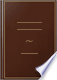Suche Bilder Maps Play YouTube News Gmail Drive Mehr »
Anmelden
 Books Bücher 1 - 10 von 121 in ... if a straight line, &c. QED PROPOSITION 29. — Theorem. If a straight line fall...... if a straight line, &c. QED PROPOSITION 29. — Theorem. If a straight line fall upon two parallel straight lines, it makes the alternate angles equal to one another ; and the exterior angle equal to the interior and opposite upon the same side ;...Elements of Geometry and Plane Trigonometry: With an Appendix, and Copious ... - Seite 30
von Sir John Leslie - 1817 - 432 Seiten
Vollansicht - Über dieses Buch## The Elements of Euclid: Viz. the First Six Books, Together with the Eleventh ...

Euclid, Robert Simson - 1806 - 518 Seiten
...the interior and position. opposite upon the same side ; and likewise the two interior angles upon the same side together equal to two right angles. Let the straight line EF fall upon the parallel straight lines AB, CD ; the alternate angles, AGH, GHD are equal to one another;...
Vollansicht - Über dieses Buch## Elements of Geometry, Geometrical Analysis, and Plane Trigonometry: With an ...

Sir John Leslie - 1809 - 493 Seiten
...in each of these three cases, the triangle ABC admits of a perfect adaptation with DEF. PROP. XXV. THEOR. If a straight line fall upon two parallel straight...interior angle EGA, and the interior angles CFG and AGF are together equal to two right angles. For suppose the straight line EFG, produced both ways from...
Vollansicht - Über dieses Buch## Elements of the Philosophy of the Human Mind, Band 2

Dugald Stewart - 1814
...asserted, for example, that ** if " one straight line falls on two other straight lines, so as to make " the two interior angles on the same side together equal to two " right angles, these two straight lines, though indefinitely pro" duced, will never meet ;"— is not the boundless...
Vollansicht - Über dieses Buch## Elements of the Philosophy of the Human Mind, Band 2

Dugald Stewart - 1814
...asserted, for example, that " if one straight line falls on two 'other straight lines, so as to make the two interior angles on the same side together equal to two righl angles, these two straight lines, though indefinitely produced, will never meet ;" — is not...
Vollansicht - Über dieses Buch## The Elements of Euclid: Viz. the First Six Books, Together with the Eleventh ...

Euclides - 1816 - 528 Seiten
...to the inte-sit10"' rior and opposite upon the same side ; and likewise the two interior angles upon the same side together equal to two right angles. „ ' . Let the straight line EF fell upon the parallel straight lines AB, CD ; the alternate angles AGH, GHD, are equal to one another...
Vollansicht - Über dieses Buch## Elements of Geometry: Containing the First Six Books of Euclid: With a ...

John Playfair - 1819 - 317 Seiten
...angle equal to the interior and opposite upon the same side ; and likewise the too interior angles upon the same side together equal to two right angles. Let the straight line EF fall upon the parallel straight lines AB, CD ; the alternate angles AGH, GHD are equal to one another...
Vollansicht - Über dieses Buch## A Popular Course of Pure and Mixed Mathematics ...: With Tables of ...

Peter Nicholson - 1825 - 372 Seiten
...angle equal to the interior and opposite upon the same side ; and likewise the two interior angles upon the same side together equal to two right angles. Let the straight line EF fall upon the parallel straight lines AB, CD ; the alternate angles AGH, GHD are equal to one another...
Vollansicht - Über dieses Buch## Elements of Geometry: With Notes

John Radford Young - 1827 - 208 Seiten
...side are together equal to two right angles. Cor. 4. Also, a line intersecting two parallels makes the two interior angles on the same side together equal to two right angles. . Cor. 5. A line perpendicular to one of two parallels is perpendicular to the other. Cor. 6. Therefore,...
Vollansicht - Über dieses Buch## The Elements of Euclid: The Errors by which Theon, Or Others, Have Long ...

Robert Simson - 1827 - 513 Seiten
...equal to the interior and opposite upon the same side .• and likewise the two interior angles upon the same side together equal to two right angles. Let the straight line EF fall upon the parallel straight lines AB, CD : the alternate angles AGH, GHD, shall be equal to...
Vollansicht - Über dieses Buch## Elements of Geometry: With Notes

John Radford Young - 1827 - 208 Seiten
...are together equal to two right angles. Cor. 4. Also, a line intersecting two parallels makes (lie two interior angles on the same side together equal to two right angles. Cor. 5. A line perpendicular to one of two parallels is perpendicular to the other. Cor. 6. Therefore,...
Vollansicht - Über dieses Buch